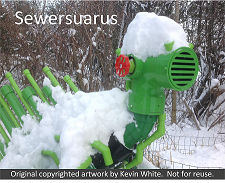# REBOL for COBOL programmers

## Right-to-left evaluation

Date written: September 25, 2012
Date revised:
Date reviewed:

## Functions of functions

It might help to reflect on high-school algebra, where the introduced the idea of a function, y = f(x). After they did that, then they introduced the idea of a function of a function, y = f(g(x)), where you evaluate g first, then put the result into f and evaluate f, and then get a final result.

## COBOL statements

COBOL statements are declarative. You tell the computer to do something, ADD, MOVE, READ, WRITE. You can have a certain amount of compound-ness in a COBOL statement. For example, you could say

```IF (data-name-1 = (data-name-2 +100))
COMPUTE data-name-3 = data-name-1 * 4
```
In a statement like that, there is some stuff going on behind the scenes, in the area of temporary data items to hold the values of various computations.

## REBOL statements

In REBOL, a statement in the language is a bunch of words. As the interpreter scans words, it evaluates them. You can think of that as meaning it replaces the words with their values. If the value of a word is, for example, a number, then that word is replaced by the number. If the word is one of REBOL's functions, then that word is replaced by the result of executing that function.

A REBOL statement is scanned from left to right. If the interpreter encounters a function word, then it knows that the function takes a certain number of parameters, so it scans to the right looking for them, and when it finds them, it then evaluates the function. For example,

```print "this is a test"
```
In this case, the "print" function needs something to print, so the interpreter scans to the right and finds the literal "this is a test" and then executes the "print" function.

Where it gets interesting and REBOL can be a bit hard to follow is in functions of functions. Remembering the COBOL example above, this is not a totally foreign notion to you, but you might not have thought of it in these terms. For example,

```print first read/lines %test.txt
```
In this statment, there are three functions, "print," "first," and "read." The interpreter evaluates from right to left. It finds the "print" function. It needs something to print. It scans ahead. It finds the "first" function. OK, it can't print yet because it has to evaluate the "first" function which requires a block of stuff to take the first thing from. So it scans ahead. Oops, another function, this time the "read" function. "Read" requires a file name, so it scans ahead again. It finds a file name, "%test.txt." Finally. So now it reads %test.txt and stores all its lines in a block. This block doesn't have a name, it is like the internal data item in the COBOL example above. Then it passes that block to the "first" function and the "first" function takes off the first item. Then it passes that first item to the "print" function, and the "print" function prints it.

This statement evaluation can get involved, and it can be a bit of a shock to the system for a person more used to COBOL programs. However, one does get used to it, and one's REBOL code can be structured in a more COBOL-like way to reduce that shock. This will be explained in later examples.